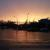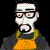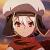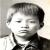如何进行C语言数组拷贝

年薪达不到25W大数据工程师、拿不到Offer全额退款->>>[code=c]
#include <stdio.h>

struct arr{
int a1;
int b1;
};

int main(void)
{
struct arr z;
z.b1 = {6,7,8,9,10};
int i;
z.a1 = z.b1;

for(i = 0; i<=4; i++)
//z.a1[i] = z.b1[i];
printf("a1[%d]=%d\n", i,z.a1[i]);

return 0;
}

[/code]

arr_op.c: In function ‘main’:
arr_op.c:11: error: expected expression before ‘{’ token
arr_op.c:13: error: incompatible types in assignment
[root @localhost workspace]#

00int main(void) {
int a = {1,2,3,4,5};
int b;
for(int i=0;i<5;i++){
*(b+i) = *(a+i);
}
for(int i=0;i<5;i++){
printf("b[%d]: %d\n",i,b[i]);
}

}

#include <stdio.h>

int main(void) {     int a = {1,2,3,4,5};     int *p = a;     for(int i=0;i<5;i++){         printf("b[%d]: %d\n",i,p[i]);     } }

0[]数组可以通过花括号初始化，但不能赋值，因此请初始化或者依次给每一个元素赋值后再进行拷贝，拷贝可以通过循环，或者memcpy内存直接拷贝
0